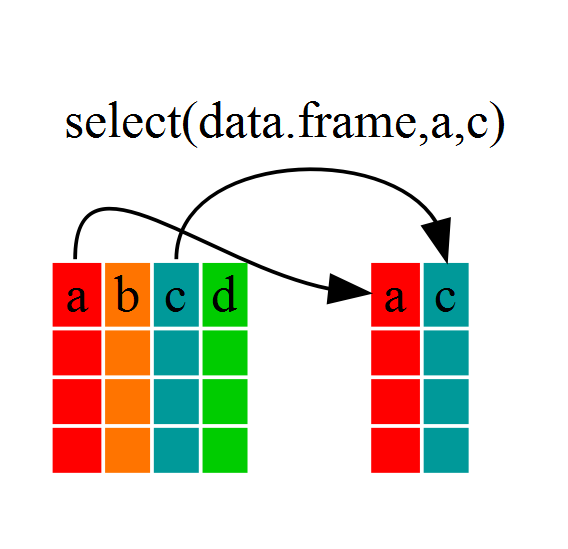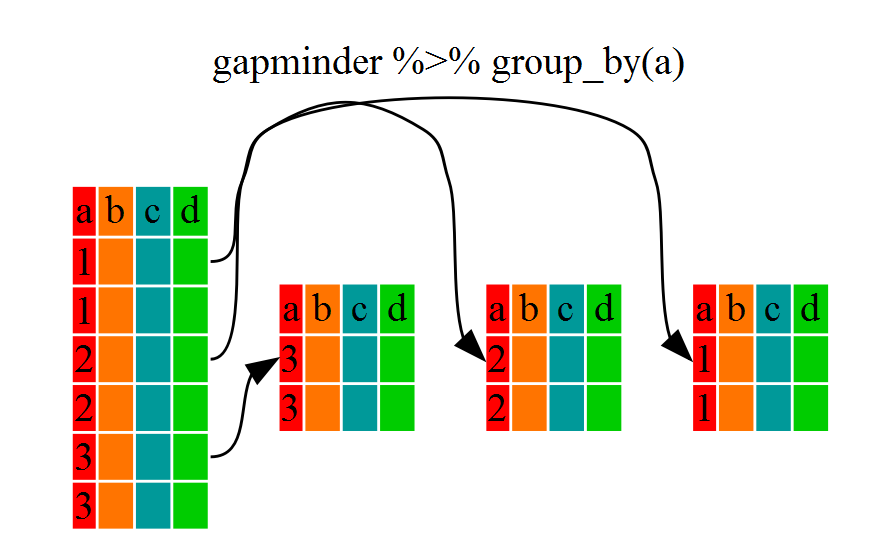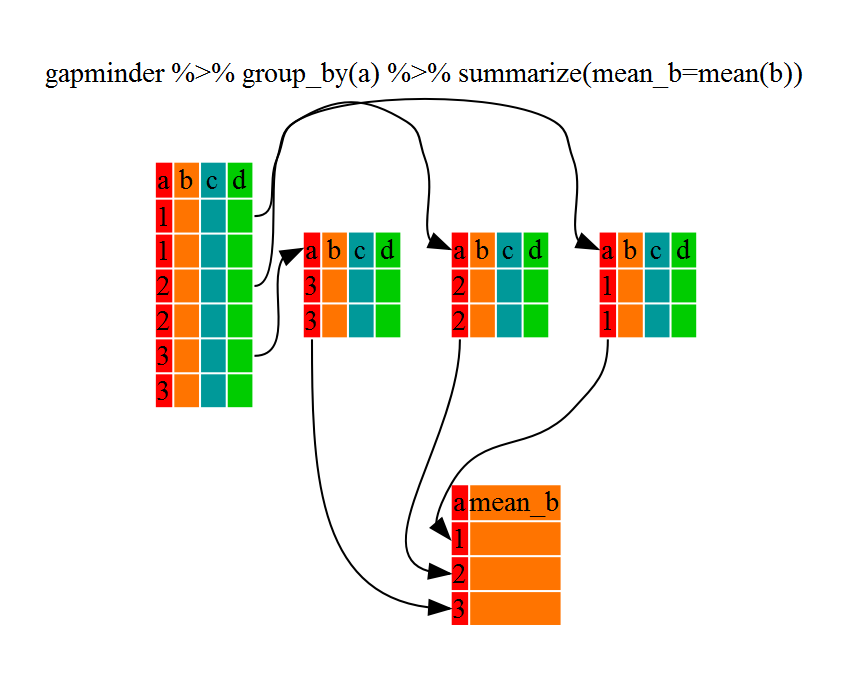# Dataframe Manipulation with dplyr

## Overview

Teaching: 40 min
Exercises: 15 min
Questions
• How can I manipulate dataframes without repeating myself?

Objectives
• To be able to use the six main dataframe manipulation ‘verbs’ with pipes in `dplyr`.

Manipulation of dataframes means many things to many researchers, we often select certain observations (rows) or variables (columns), we often group the data by a certain variable(s), or we even calculate summary statistics. We can do these operations using the normal base R operations:

``````mean(gapminder[gapminder\$continent == "Africa", "gdpPercap"])
``````
`````` 2193.755
``````
``````mean(gapminder[gapminder\$continent == "Americas", "gdpPercap"])
``````
`````` 7136.11
``````
``````mean(gapminder[gapminder\$continent == "Asia", "gdpPercap"])
``````
`````` 7902.15
``````

But this isn’t very nice because there is a fair bit of repetition. Repeating yourself will cost you time, both now and later, and make your code bulky and hard to read. But worse yet, it can potentially introduce some nasty bugs.

## The `dplyr` package

Luckily, the `dplyr` package provides a number of very useful functions for manipulating dataframes in a way that will reduce the above repetition, reduce the probability of making errors, and probably even save you some typing. As an added bonus, you might even find the `dplyr` grammar easier to read.

Here we’re going to cover 5 of the most commonly used functions as well as using pipes (`%>%`) to combine them.

1. `select`
2. `filter`
3. `group_by`
4. `summarize`
5. `mutate`

If you have have not installed this package earlier, please do so now:

``````install.packages('dplyr')
``````

``````library(dplyr)
``````

## Using select

If, for example, we wanted to move forward with only a few of the variables in our dataframe we could use the `select` function. This will keep only the variables you select.

``````year_country_gdp <- select(gapminder,year,country,gdpPercap)
``````If we open up `year_country_gdp` we’ll see that it only contains the year, country and gdpPercap. Above we used ‘normal’ grammar, but the strengths of `dplyr` lie in combining several functions using pipes. Since the pipes grammar is unlike anything we’ve seen in R before, let’s repeat what we’ve done above using pipes.

``````year_country_gdp <- gapminder %>% select(year,country,gdpPercap)
``````

To help you understand why we wrote that in that way, let’s walk through it step by step. First we summon the gapminder dataframe and pass it on, using the pipe symbol `%>%`, to the next step, which is the `select` function. In this case we don’t specify which data object we use in the `select` function since in gets that from the previous pipe. Fun Fact: There is a good chance you have encountered pipes before in the shell. In R, a pipe symbol is `%>%` while in the shell it is `|` but the concept is the same!

## Using filter

If we now wanted to move forward with the above, but only with European countries, we can combine `select` and `filter`

``````year_country_gdp_euro <- gapminder %>%
filter(continent=="Europe") %>%
select(year,country,gdpPercap)
``````

## Tip:

The line breaks in our commands do not need to be in specific locations. They can be made wherever necessary to keep your code neat and make it easier to read. Some people place them to keep the lines roughly and equal length, while others put a single option on each line. The benefit of this approach is that you can use in line comments to remind you what each option does. Whichever approach you use, remember to keep the `+` at the end of the line so R knows that your command continues on the next line.

As with last time, first we pass the gapminder dataframe to the `filter` function, then we pass the filtered version of the gapminder dataframe to the `select` function. Note: The order of operations is very important in this case. If we used ‘select’ first, filter would not be able to find the variable continent since we would have removed it in the previous step.

## Challenge 1

Write a single command (which can span multiple lines and includes pipes) that will produce a dataframe that has the African values for `lifeExp`, `country` and `year`, but not for other Continents. How many rows does your dataframe have and why?

## Solution to Challenge 1

``````year_country_lifeExp_Africa <- gapminder %>%
filter(continent=="Africa") %>%
select(year,country,lifeExp)
``````

We can check the number of rows in our new dataframe `year_country_lifeExp_Africa` by using the `ncol` command:

``````nrow(year_country_lifeExp_Africa)
``````
`````` 624
``````

## Using group_by and summarize

Now, we were supposed to be reducing the error prone repetitiveness of what can be done with base R, but up to now we haven’t done that since we would have to repeat the above for each continent. Instead of `filter`, which will only pass observations that meet your criteria (in the above: `continent=="Europe"`), we can use `group_by`, which will essentially use every unique criteria that you could have used in `filter`.

``````str(gapminder)
``````
``````'data.frame':	1704 obs. of  6 variables:
\$ country  : Factor w/ 142 levels "Afghanistan",..: 1 1 1 1 1 1 1 1 1 1 ...
\$ year     : int  1952 1957 1962 1967 1972 1977 1982 1987 1992 1997 ...
\$ pop      : num  8425333 9240934 10267083 11537966 13079460 ...
\$ continent: Factor w/ 5 levels "Africa","Americas",..: 3 3 3 3 3 3 3 3 3 3 ...
\$ lifeExp  : num  28.8 30.3 32 34 36.1 ...
\$ gdpPercap: num  779 821 853 836 740 ...
``````
``````str(gapminder %>% group_by(continent))
``````
``````Classes 'grouped_df', 'tbl_df', 'tbl' and 'data.frame':	1704 obs. of  6 variables:
\$ country  : Factor w/ 142 levels "Afghanistan",..: 1 1 1 1 1 1 1 1 1 1 ...
\$ year     : int  1952 1957 1962 1967 1972 1977 1982 1987 1992 1997 ...
\$ pop      : num  8425333 9240934 10267083 11537966 13079460 ...
\$ continent: Factor w/ 5 levels "Africa","Americas",..: 3 3 3 3 3 3 3 3 3 3 ...
\$ lifeExp  : num  28.8 30.3 32 34 36.1 ...
\$ gdpPercap: num  779 821 853 836 740 ...
- attr(*, "vars")=List of 1
..\$ : symbol continent
- attr(*, "drop")= logi TRUE
- attr(*, "indices")=List of 5
..\$ : int  24 25 26 27 28 29 30 31 32 33 ...
..\$ : int  48 49 50 51 52 53 54 55 56 57 ...
..\$ : int  0 1 2 3 4 5 6 7 8 9 ...
..\$ : int  12 13 14 15 16 17 18 19 20 21 ...
..\$ : int  60 61 62 63 64 65 66 67 68 69 ...
- attr(*, "group_sizes")= int  624 300 396 360 24
- attr(*, "biggest_group_size")= int 624
- attr(*, "labels")='data.frame':	5 obs. of  1 variable:
..\$ continent: Factor w/ 5 levels "Africa","Americas",..: 1 2 3 4 5
..- attr(*, "vars")=List of 1
.. ..\$ : symbol continent
..- attr(*, "drop")= logi TRUE
``````

You will notice that the structure of the dataframe where we used `group_by` (`grouped_df`) is not the same as the original `gapminder` (`data.frame`). A `grouped_df` can be thought of as a `list` where each item in the `list`is a `data.frame` which contains only the rows that correspond to the a particular value `continent` (at least in the example above).## Using summarize

The above was a bit on the uneventful side because `group_by` is much more exciting in conjunction with `summarize`. This will allow use to create new variable(s) by using functions that repeat for each of the continent-specific data frames. That is to say, using the `group_by` function, we split our original dataframe into multiple pieces, then we can run functions (e.g. `mean` or `sd`) within `summarize`.

``````gdp_bycontinents <- gapminder %>%
group_by(continent) %>%
summarize(mean_gdpPercap=mean(gdpPercap))
gdp_bycontinents
``````
``````# A tibble: 5 x 2
continent mean_gdpPercap
<fctr>          <dbl>
1    Africa       2193.755
2  Americas       7136.110
3      Asia       7902.150
4    Europe      14469.476
5   Oceania      18621.609
``````That allowed us to calculate the mean gdpPercap for each continent, but it gets even better.

## Challenge 2

Calculate the average life expectancy per country. What is the longest average life expectancy and the shortest life expectancy?

## Solution to Challenge 2

First let’s build a dataframe with a summary of the average life expectancy per country:

``````lifeExp_bycountry <- gapminder %>%
group_by(country) %>%
summarize(mean_lifeExp=mean(lifeExp))
``````

Now that we have the data we need, we can use the `min` and `max` commands to determine which country had the longest and shortest life expectancy:

``````min(lifeExp_bycountry\$mean_lifeExp)
``````
`````` 36.76917
``````
``````max(lifeExp_bycountry\$mean_lifeExp)
``````
`````` 76.51142
``````

The function `group_by` allows us to group by multiple variables. Let’s group by `year` and `continent`.

``````gdp_bycontinents_byyear <- gapminder %>%
group_by(continent,year) %>%
summarize(mean_gdpPercap=mean(gdpPercap))
``````

That is already quite powerful, but it gets even better! You’re not limited to defining 1 new variable in `summarize`.

``````gdp_pop_bycontinents_byyear <- gapminder %>%
group_by(continent,year) %>%
summarize(mean_gdpPercap=mean(gdpPercap),
sd_gdpPercap=sd(gdpPercap),
mean_pop=mean(pop),
sd_pop=sd(pop))
``````

## Using mutate

We can also create new variables prior to (or even after) summarizing information using `mutate`.

``````gdp_pop_bycontinents_byyear <- gapminder %>%
mutate(gdp_billion=gdpPercap*pop/10^9) %>%
group_by(continent,year) %>%
summarize(mean_gdpPercap=mean(gdpPercap),
sd_gdpPercap=sd(gdpPercap),
mean_pop=mean(pop),
sd_pop=sd(pop),
mean_gdp_billion=mean(gdp_billion),
sd_gdp_billion=sd(gdp_billion))
``````

## Challenge 3

Calculate the average life expectancy in 2002 for each continent.

## Solution to Challenge 3

``````lifeExp_bycontinents <- gapminder %>%
filter(year==2002) %>%
group_by(continent) %>%
summarize(mean_lifeExp=mean(lifeExp))
``````

Modify your code from Challenge 3 to randomly select 2 countries from each continent before calculating the average life expectancy and then arrange the continent names in reverse order.

Hint: Use the `dplyr` functions `arrange` and `sample_n`, they have similar syntax to other dplyr functions. Be sure to check out the help documentation for the new functions by typing `?arrange` or `?sample_n` if you run into difficulties.

## Solution to Challenge 4

``````lifeExp_2countries_bycontinents <- gapminder %>%
filter(year==2002) %>%
group_by(continent) %>%
summarize(mean_lifeExp=mean(lifeExp)) %>%
sample_n(2) %>%
arrange(desc(mean_lifeExp))
``````

## Key Points

• Use the `dplyr` package to manipulate dataframes.

• Use `select` to choose variables from a dataframe.

• Use `filter` to choose data based on values.

• Use `group_by` and `summarize` to work with subsets of data.

• Use `mutate` to create new variables.